# 出生日期输入输出

20130225

year=2013
month=02
date=25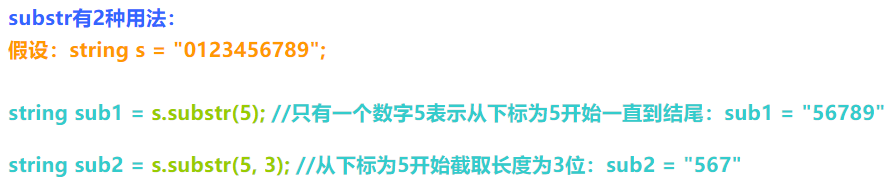#include <bits/stdc++.h>
using namespace std;
int main()
{
string s;
cin>>s;
cout<<"year="<<s.substr(0,4)<<endl
<<"month="<<s.substr(4,2)<<endl
<<"date="<<s.substr(6,2);
return 0;
}


# 字符金字塔

1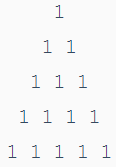#include<bits/stdc++.h>
using namespace std;
int main()
{
char c;
cin>>c;
cout<<"    "<<c<<endl
<<"   "<<c<<" "<<c<<endl
<<"  "<<c<<" "<<c<<" "<<c<<endl
<<" "<<c<<" "<<c<<" "<<c<<" "<<c<<endl
<<c<<" "<<c<<" "<<c<<" "<<c<<" "<<c;
return 0;
}


# 大小写转换

A
B

a
b

#include <stdio.h>
int main()
{
char ch;
while((ch=getchar())!=EOF)
{
if(ch>='A'&&ch<='Z')    ch+=32;
putchar(ch);
}
}
/*

#include <stdio.h>
#include <ctype.h>
int main(void)
{
char ch;
while(scanf("%c", &ch) == 1)
{
putchar(tolower(ch));
getchar();
}
return 0;
}
*/


# KiKi和酸奶

BoBo买了一箱酸奶，里面有n盒未打开的酸奶，KiKi喜欢喝酸奶，第一时间发现了酸奶。KiKi每h分钟能喝光一盒酸奶，并且KiKi在喝光一盒酸奶之前不会喝另一个，那么经过m分钟后还有多少盒未打开的酸奶？

8 5 16

4

#include <bits/stdc++.h>
using namespace std;

int main()
{
int n=0, h=0, m=0;
//循环读入n，h，m
while(scanf("%d %d %d", &n, &h, &m) != EOF)
{
//判断是否能够整除，如果可以输出 n-m/h），反之减1
if(m%h == 0)    printf("%d\n", n - (m / h));
else    printf("%d\n", n-(m/h)-1);
}
return 0;
}


# 衡量人体胖瘦程度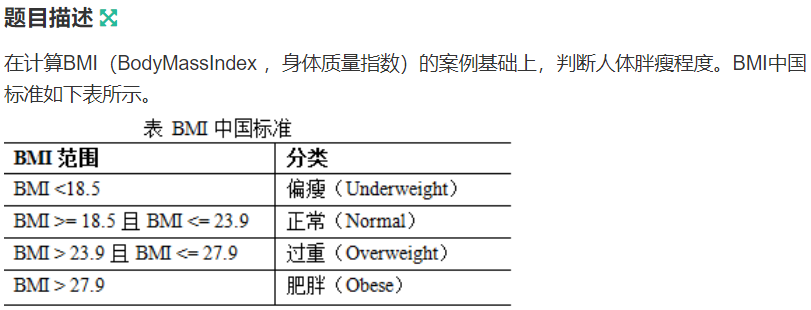80 170
60 170
90 160
50 185

Overweight
Normal
Obese
Underweight

#include <bits/stdc++.h>
using namespace std;

int main()
{
int weight=0, height=0;
float BMI=0.f;
//循环读入体重和身高
while(scanf("%d %d", &weight, &height) != EOF)
{
//根据公式计算BMI
BMI = weight / (height/100.f * height/100.f);
//使用if else嵌套判断BMI所在的区间，输出即可
if(BMI < 18.5f)     printf("Underweight\n");
else if(BMI >=18.5f && BMI <= 23.9f)    printf("Normal\n");
else if(BMI > 23.9f && BMI <= 27.9f)     printf("Overweight\n");
else     printf("Obese\n");
}
return 0;
}


# 获得月份天数

KiKi想获得某年某月有多少天，请帮他编程实现。输入年份和月份，计算这一年这个月有多少天。

2008 2

29

#include <stdio.h>
int main()
{
int a,b;
while(scanf("%d%d",&a,&b)!=EOF)
{
if(b==1||b==3||b==5||b==7||b==8||b==10||b==12)    b=31;
else if(b==2) {
b=28;
if((a%400==0)||(a%4==0&&a%100!=0))    b++;
}
else     b=30;
printf("%d\n",b);
}
}


# 竞选社长

ABBABBAAB0

B

#include <bits/stdc++.h>
using namespace std;

int main()
{
char a;
int cc1 = 0, cc2 = 0;

scanf("%s", a);
int n = strlen(a);
for (int i=0; i<n-1; i++) {
if (a[i]=='A')  cc1++;
else  cc2++;
}
if(cc1>cc2)  puts("A");
else if(cc1<cc2)  puts("B");
else  puts("E");
}


# K形图案

KiKi学习了循环，BoBo老师给他出了一系列打印图案的练习，该任务是打印用“*”组成的K形图案。

2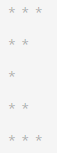3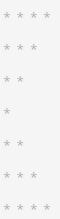4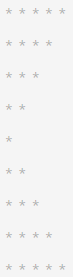#include <stdio.h>
int main()
{
int i,j,k,n;
while(scanf("%d",&n)!=EOF)
{
for(i=1; i<=n; i++)
{
printf("* ");
for(j=1;j<=n-i+1;j++)    printf("* ");
for(k=0; k<i-1; k++)    printf(" ");
printf("\n");
}
for(i=1; i<=n+1; i++)
{
printf("*");
for(j=1; j<=i-1; j++)    printf(" *");
for(k=0; k<=n-i+1; k++)    printf(" ");
printf("\n");
}
}
return 0;
}
/*

#include <bits/stdc++.h>
using namespace std;

int main()
{
int n;
while(cin>>n)
{
for(int i=n+1; i>=1; i--)
{
for(int j=1; j<=i ;j++)  cout<<"*"<<" ";
cout<<endl;
}
for(int i=2; i<=n+1; i++)
{
for(int j=1; j<=i; j++)  cout<<"*"<<" ";
cout<<endl;
}
}
return 0;
}
*/


# 正斜线形图案

KiKi学习了循环，BoBo老师给他出了一系列打印图案的练习，该任务是打印用星号组成的正斜线形图案。

4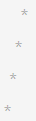5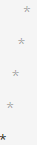#include <bits/stdc++.h>
using namespace std;

int main() {
int n = 0;
while(scanf("%d", &n) != EOF)
{
int space = n-1;
for(int i=0; i<n; ++i)
{
for(int j=0; j<space; ++j)    printf(" ");
printf("*");
for(int k=0; k<i; ++k)    printf(" ");
printf("\n");
space--;
}
}
return 0;
}


# X形图案

KiKi学习了循环，BoBo老师给他出了一系列打印图案的练习，该任务是打印用星号组成的X形图案。

5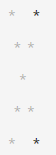6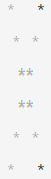#include <bits/stdc++.h>
using namespace std;

int main() {
int n;
while (scanf("%d", &n) != EOF)
{
for(int i=0; i<n; ++i)
{
for(int j=0; j<n; ++j)
{
if(i==j)    printf("*");
else if(j == n-i-1)     printf("*");
else     printf(" ");
}
printf("\n");
}
}
return 0;
}

08-101336
06-1312906-22337
05-03564
08-08472
03-251602
05-106037
08-10113
07-2411
03-20346